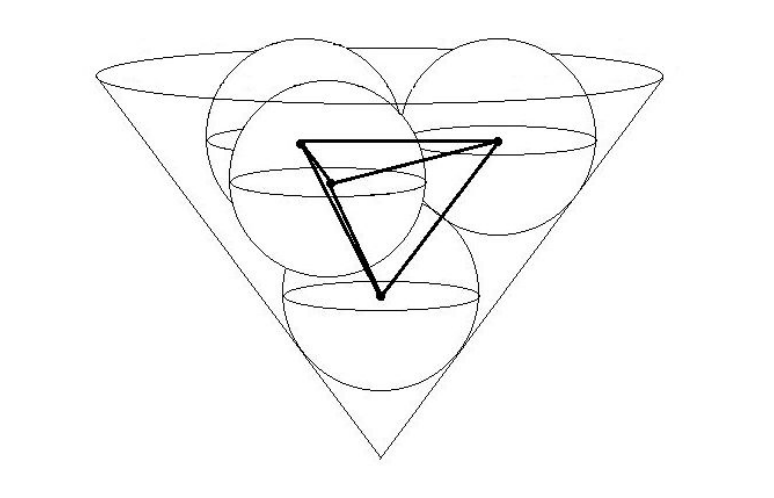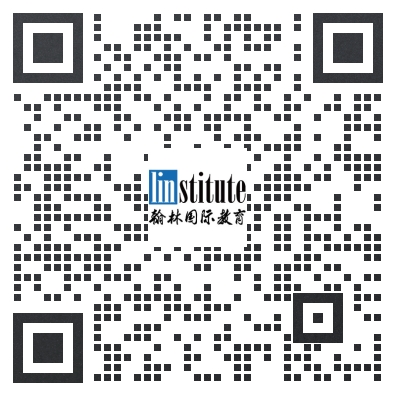# 紫色彗星数学竞赛真题（2017年高中组）

PURPLE COMET! MATH MEET April 2017

HIGH SCHOOL - PROBLEMS

Copyright Titu Andreescu and Jonathan Kane

Problem 1

Paul starts at 1 and counts by threes: 1, 4, 7, 10, . . . . At the same time and at the same speed, Penny counts backwards from 2017 by fifives: 2017, 2012, 2007, 2002, . . . . Find the one number that both Paul and Penny count at the same time.

Problem 2

The fifigure below shows a large square divided into 9 congruent smaller squares. A shaded square bounded by some of the diagonals of those smaller squares has area 14. Find the area of the large square.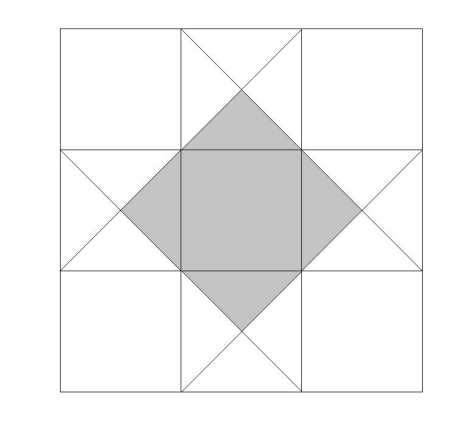Problem 3

When Phil and Shelley stand on a scale together, the scale reads 151 pounds. When Shelley and Ryan stand on the same scale together, the scale reads 132 pounds. When Phil and Ryan stand on the same scale together, the scale reads 115 pounds. Find the number of pounds Shelley weighs.

Problem 4

Find the least positive integer m such that lcm(15, m) = lcm(42, m). Here lcm(a, b) is the least common multiple of a and b.

Problem 5

A store had 376 chocolate bars. Min bought some of the bars, and Max bought 41 more of the bars than Min bought. After that, the store still had three times as many chocolate bars as Min bought. Find the number of chocolate bars that Min bought.

Problem 6

For some constant k the polynomial p(x) = 3x2 + kx + 117 has the property that p(1) = p(10). Evaluate p(20).

Problem 7

Consider an alphabetized list of all the arrangements of the letters in the word BETWEEN. Then BEEENTW would be in position 1 in the list, BEEENWT would be in position 2 in the list, and so forth. Find the position that BETWEEN would be in the list.

Problem 8

Find the number of trailing zeros at the end of the base-10 representation of the integer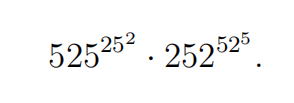Problem 9

The diagram below shows △ABC with point D on side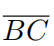. Three lines parallel to sidedivide segment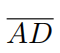into four equal segments. In the triangle, the ratio of the area of the shaded region to the area of the

unshaded region is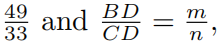where m and n are relatively prime positive integers. Find m + n.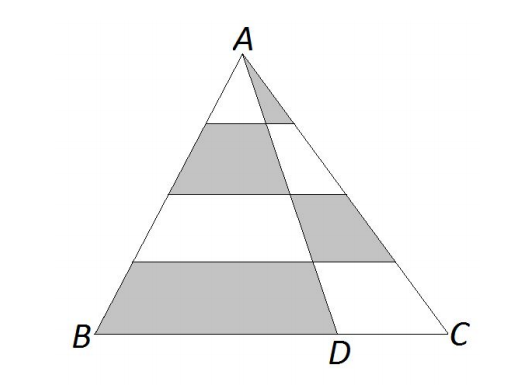Problem 10

Find the number of positive integers less than or equal to 2017 that have at least one pair of adjacent digits that are both even. For example, count the numbers 24, 1862, and 2012, but not 4, 58, or 1276.

Problem 11

Dave has a pile of fair standard six-sided dice. In round one, Dave selects eight of the dice and rolls them. He calculates the sum of the numbers face up on those dice to get r1. In round two, Dave selects r1 dice and rolls them. He calculates the sum of the numbers face up on those dice to get r2. In round three, Dave selects r2 dice and rolls them. He calculates the sum of the numbers face up on those dice to get r3. Find he expected value of r3.

Problem 12

Let P be a polynomial satisfying P(x + 1) + P(x 1) = x3 for all real numbers x. Find the value of P(12).

Problem 13

Let ABCDE be a pentagon with area 2017 such that four of its sides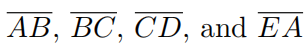have integer

length. Suppose that ∠A = ∠B = ∠C = 90°, AB = BC, and CD = EA. The maximum possible perimeter

of ABCDE is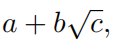where a, b, and c are integers and c is not divisible by the square of any prime.

Find a + b + c.

Problem 14

Find the sum of all integers n for which n 3 and n2 + 4 are both perfect cubes.

Problem 15

For real numbers a, b, and c the polynomial p(x) = 3x7 291x6 + ax5 + bx4 + cx2 + 134x 2 has 7 real roots whose sum is 97. Find the sum of the reciprocals of those 7 roots.

Problem 16

Let a1 = 1 +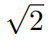and for each n 1 defifine an+1 = 2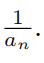Find the greatest integer less than or equal to

the product a1a2a3 · · · a200.

Problem 18

In the 3-dimensional coordinate space fifind the distance from the point (36, 36, 36) to the plane that passes through the points (336, 36, 36), (36, 636, 36), and (36, 36, 336).

Problem 19

Find the greatest integer n < 1000 for which 4n3 3n is the product of two consecutive odd integers.

Problem 20

Let a be a solution to the equation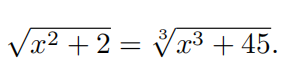Evaluate the ratio of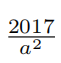to a2 15a + 2.

Problem 21

The diagram below shows a large circle. Six congruent medium-sized circles are each internally tangent to the large circle and tangent to two neighboring medium-sized circles. Three congruent small circles are mutually tangent to one another and are each tangent to two medium-sized circles as shown. The ratio of the area of the large circle to the area of one of the small circles can be written as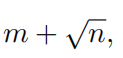where m and are positive integers. Find m + n.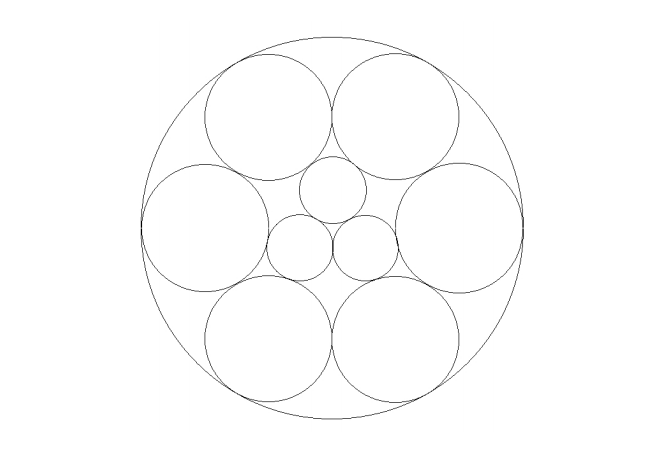Problem 22

Find the number of functions f that map the set {1, 2, 3, 4} into itself such that the range of the function f(x) is the same as the range of the function f(f(x)).

Problem 23

The familiar 3-dimensional cube has 6 2-dimensional faces, 12 1-dimensional edges, and 8 0-dimensional vertices. Find the number of 9-dimensional sub-subfaces in a 12-dimensional cube.

Problem 24

Eight red boxes and eight blue boxes are randomly placed in four stacks of four boxes each. The probability that exactly one of the stacks consists of two red boxes and two blue boxes is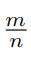, where m and n are relatively prime positive integers. Find m + n.

Problem 25

Leaving his house at noon, Jim walks at a constant rate of 4 miles per hour along a 4 mile square route returning to his house at 1 PM. At a randomly chosen time between noon and 1 PM, Sally chooses a random location along Jim’s route and begins running at a constant rate of 7 miles per hour along Jim’s route in the same direction that Jim is walking until she completes one 4 mile circuit of the square route. The probability that Sally runs past Jim while he is walking is given by, where m and n are relatively prime positive integers. Find m + n.

Problem 26

The incircle of 4 ABC is tangent to sides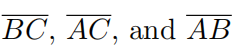at D, E, and F, respectively. Point G is the intersection of lines AC and DF as shown. The sides of 4 ABC have lengths AB = 73, BC = 123, and AC = 120. Find the length EG.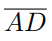Problem 27

Find the minimum value of 4(x2 + y2 + z2 + w2 ) + (xy 7)2 + (yz 7)2 + (zw 7)2 + (wx 7)2 as x, y, z, and w range over all real numbers.

Problem 28

Let Tk = k(k2 +1) be the kth triangular number. The infifinite series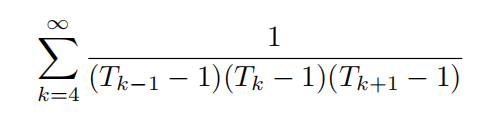has the value, where m and n are relatively prime positive integers. Find m + n.

Problem 29

Find the number of three-element subsets of {1, 2, 3, . . . , 13} that contain at least one element that is a multiple of 2, at least one element that is a multiple of 3, and at least one element that is a multiple of 5 such as {2, 3, 5} or {6, 10, 13}.

Problem 30

A container is shaped like a right circular cone with base diameter 18 and height 12. The vertex of the container is pointing down, and the container is open at the top. Four spheres, each with radius 3, are placed inside the container as shown. The fifirst sphere sits at the bottom and is tangent to the cone along a circle. The second, third, and fourth spheres are placed so they are each tangent to the cone and tangent to the fifirst sphere, and the second and fourth spheres are each tangent to the third sphere. The volume of the tetrahedron whose vertices are at the centers of the spheres is K. Find K2.